Introduction to Hydrology (Geog 3511)

Fall 2012

Assignment 11: Streamflow Analysis

 Section Lab Assigned Due 012,013 Tuesday Tuesday, Dec 4 Friday, Dec 7 011 Thursday Thursday, Dec 6 Monday, Dec 10

40 possible points.

CITE SOURCES OF EQUATIONS USED.

WRITE THE QUESTION AT THE START OF EACH ANSWER.

## Manning's Equation

Manning's equation is commonly used to calculate the mean channel velocity of a stream. Manning's equation can be written as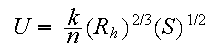where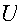is the discharge velocity (in m/s),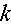is the unit conversion factor,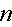is the Manning's n coefficient,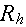is the hydraulic radius (in meters), and S is the slope (in meter/meter).

The conversion factor,, is used because the equation is dimensionally inhomogeneous. The value ofdepends on the choice of units used forand. Because we are using standard metric units (meters forand meters/sec for) the value ofis conveniently 1.0. If you consider the units of, you would use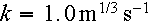.

Manning'sis a dimensionless number that characterizes the resistance of the channel to flow. Higher values ofindicate greater resistance. Dingman presents a table of typical Manning'svalues (Table 9-6). Also there is a USGS website that lists the n values of actual rivers. http://wwwrcamnl.wr.usgs.gov/sws/fieldmethods/Indirects/nvalues/

The hydraulic radius,, is the cross-sectional area of the stream divided by the wetted perimeter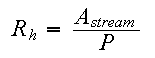River channels are commonly much wider than they are deep. Because of this, we sometimes approximate the wetted perimeter of the channel,, by the width of the channel.Because area has units of square meters and the perimeter has units of meters, the hydraulic radius also has units of meters.

### Effect of channel bottom

Bottom roughness of a stream has a large effect on the velocity of water in the channel. Lets solve Mannings equation for mean channel velocity for a smooth sand bed (n=0.025) and a very coarse weedy bed (n=0.075).

Use the following table to answer questions 1 through 4:

 Channel 1 Channel 2 cross-sectional area 12.0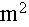12.0average stream depth 1.5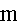1.5channel bottom smooth sand weedy bed slope 0.00060.0006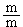Q1) What is the hydraulic radius?

Q2) What is mean stream velocity for the channel with a smooth sandy bed?

Q3) What is mean stream velocity for the channel with a weedy bed?

Q4) What is the ratio of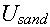to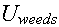?

### Effect of slope

Q5) Using the approximation that the channel width is much greater than the depth, rearrange Manning's equation to solve for stream depth. Based on the equation, what effect will an increased slope have on the depth of water? (Note: do not use numbers to answer this question)

Use the following table to answer questions 6 and 7:

 Channel 1 Channel 2 mean channel velocity 2.0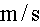2.0average stream width 1010channel bottom smooth sand smooth sand slope 0.00060.0016Q6) What is mean stream depth for the channel with a lesser slope?

Q7) What is mean stream depth for the channel with a greater slope?
Q8) Are the answers from questions 6 and 7 realistic in terms of the assumed width/depth ratio?

### Effect of stream depth

We can rewrite Manning's equation to calculate the discharge as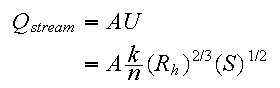Q9) Using the assumption that the channel width is much greater than the depth, rewrite the preceding equation so that it is in the formwhereis the exponent applied to the depth of the stream. (Note: remember that the hydraulic radius is a function of depth)

Q10) Based on the equation derived in Q9, what effect will an increased depth have on the discharge of the stream? What is the value of the exponent? Graph the equation, with the depth of the stream on the x-axis and discharge on the y-axis.